# TrigCalc · Trigonometry Calcul

4.2
9 reviews
1K+Everyone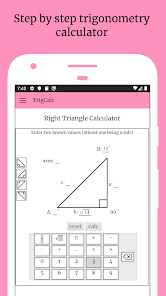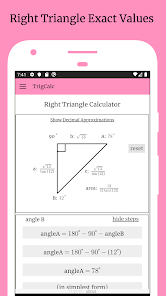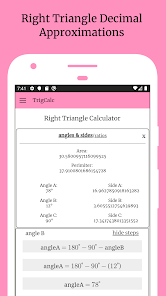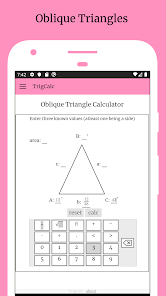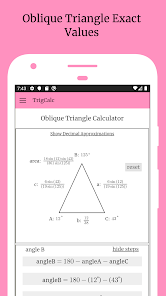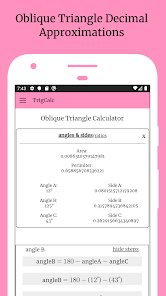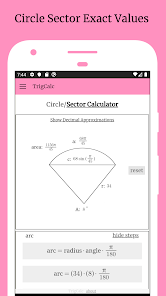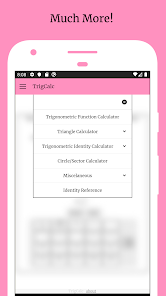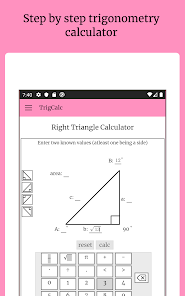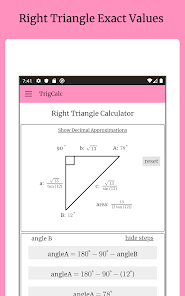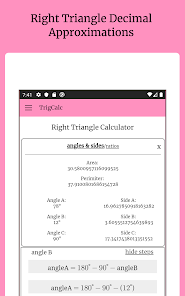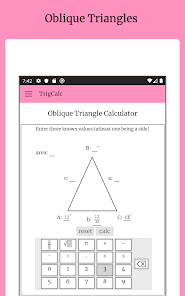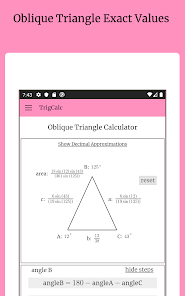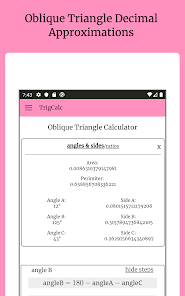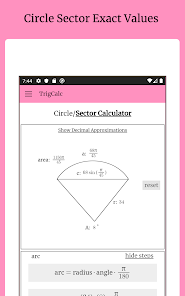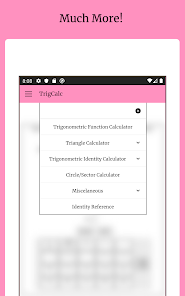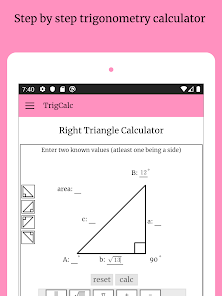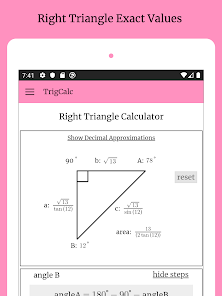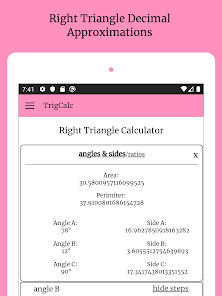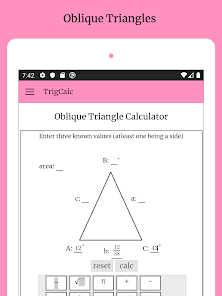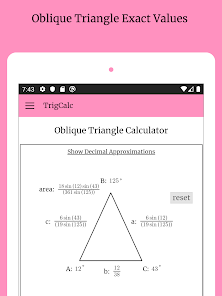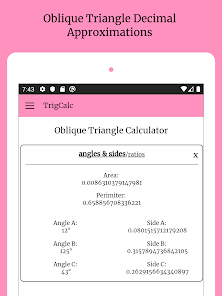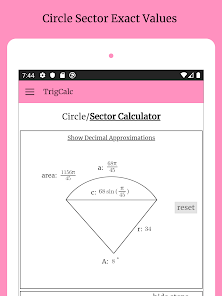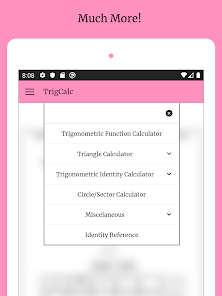Trigcalc trigonometry calculator helps to teach & solve math, Triangle calculator is also created to solve and teach trigonometry in the simplest way possible. It is also the most popular one. All calculators are returns step by step explanations for all of its calculators. There is a right triangle, It is represented with a rotatable right triangle with math fields located in their corresponding locations. All math fields support the use of exponents, radicals, pi, multiplication, division, and decimals. To make a right triangle calculation, enter two values with one being a side and tap the calc button. After about two seconds worth of load time, Trigcalc displays the exact values of all missing sides and angles in the math fields with the steps for each value below. The oblique triangle works in a similar way. It uses a different set of formulas for calculations and may display two solutions. It also does not rotate. Another popular is its coterminal angle. The coterminal angle is displayed with a drop down option list requesting either the least positive or least negative angle coterminal with a certain angle in either degree or radian form. After selecting from the option list and tapping the calc button, returns the exact value, decimal approximation, and calculation process for the coterminal angle. Identity calculators include double angle, half angle, power reduction, sum difference, sum to product, and product to sum. Identity calculations require a chosen identity, a given function, a given ratio, and a given quadrant. The solution consists of the exact value of tan2u and steps including how sinu in quadrant one can be used to find tan2u. While it is not as popular Sector is very useful. Sector calculations contain a sector image and math fields just like triangle calculations. Sectors require two values as at least one being radius or angle and provide all values. It also allows you to swap to its circle that only requires one value. The function calculator allows you to get the value of all trig functions given any value. Each function returns a solution to sixteen decimal precision. Supplementary angles are angles whose sum equals one hundred and eighty degrees. Supplementary takes in one angle in either degree or radian form and sends back the corresponding supplementary angle with is one hundred and eighty minus the given value. Complementary angles are angles whose sum adds up to ninety degrees. complementary works very similarly to its supplementary by returning ninety minus the given value with steps and exact values. For every exact value in Trigcalc, a decimal approximation is also available. All of the decimal approximations have sixteen digit precision ex 5.9718346348117407. The trigonometry calculator is aimed for simplicity and accuracy.
Updated on
Mar 20, 2020

## Data safetyNo information available
4.2
9 reviews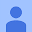February 28, 2020
the app is beautiful and functional as advertised.
5 people found this review helpful
TrigCalc
February 29, 2020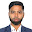Abu Darda
June 16, 2021
Good App. But problem is offline not working.
1 person found this review helpful